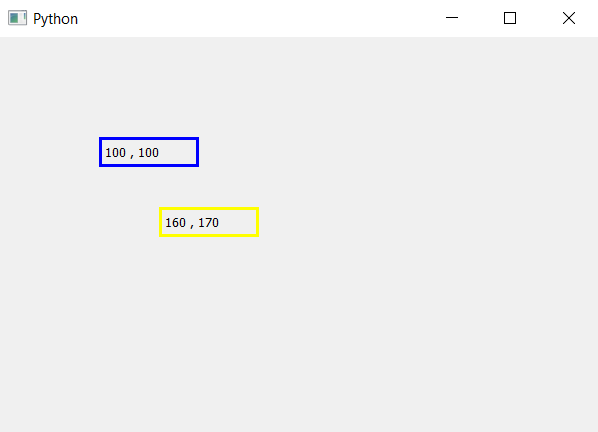# PyQt5 – How to get Label coordinates ?

The main window in PyQt5 is like graph it has x-axis and y-axis, all the widgets are positioned according to their x, y co-ordinates. When we create label is formed at the top left corner, the op left co-ordinates are (0, 0), we can move the label using `move()` method.
In this article, we will see how to get the co-ordinates of label. In order to do so we will use `x()` method for x co-ordinate and `y()` method for y co-ordinate.

Syntax :

```x_position = label.x()
y_position =label.y()
```

Argument : Both method takes no argument.

Return : Both returns integer.

Code :

 `# importing the required libraries ` ` `  `from` `PyQt5.QtCore ``import` `*`  `from` `PyQt5.QtGui ``import` `*`  `from` `PyQt5.QtWidgets ``import` `*`  `import` `sys ` ` `  ` `  `class` `Window(QMainWindow): ` ` `  ` `  `    ``def` `__init__(``self``): ` `        ``super``().__init__() ` ` `  ` `  `        ``# set the title ` `        ``self``.setWindowTitle(``"Python"``) ` ` `  `        ``# setting  the geometry of window ` `        ``self``.setGeometry(``60``, ``60``, ``600``, ``400``) ` ` `  ` `  `        ``# creating a label widget ` `        ``self``.label_1 ``=` `QLabel(``self``) ` ` `  `        ``# moving position ` `        ``self``.label_1.move(``100``, ``100``) ` ` `  `        ``# setting up the border ` `        ``self``.label_1.setStyleSheet(``"border :3px solid blue;"``) ` ` `  `        ``# getting x and y co-ordinates ` `        ``x ``=` `str``(``self``.label_1.x()) ` `        ``y ``=` `str``(``self``.label_1.y()) ` ` `  `        ``# setting label text ` `        ``self``.label_1.setText(x``+``", "``+` `y) ` ` `  `        ``# creating a label widget ` `        ``self``.label_2 ``=` `QLabel(``self``) ` ` `  `        ``# moving position ` `        ``self``.label_2.move(``160``, ``170``) ` ` `  `        ``# setting up the border ` `        ``self``.label_2.setStyleSheet(``"border :3px solid yellow;"``) ` ` `  `        ``# getting x and y co-ordinates ` `        ``x ``=` `str``(``self``.label_2.x()) ` `        ``y ``=` `str``(``self``.label_2.y()) ` ` `  `        ``# setting label text ` `        ``self``.label_2.setText(x ``+` `", "` `+` `y) ` ` `  `        ``# show all the widgets ` `        ``self``.show() ` ` `  ` `  `# create pyqt5 app ` `App ``=` `QApplication(sys.argv) ` ` `  `# create the instance of our Window ` `window ``=` `Window() ` `# start the app ` `sys.exit(App.``exec``()) `

Output :Attention geek! Strengthen your foundations with the Python Programming Foundation Course and learn the basics.

To begin with, your interview preparations Enhance your Data Structures concepts with the Python DS Course.

My Personal Notes arrow_drop_upCheck out this Author's contributed articles.

If you like GeeksforGeeks and would like to contribute, you can also write an article using contribute.geeksforgeeks.org or mail your article to contribute@geeksforgeeks.org. See your article appearing on the GeeksforGeeks main page and help other Geeks.

Please Improve this article if you find anything incorrect by clicking on the "Improve Article" button below.

Article Tags :

Be the First to upvote.

Please write to us at contribute@geeksforgeeks.org to report any issue with the above content.